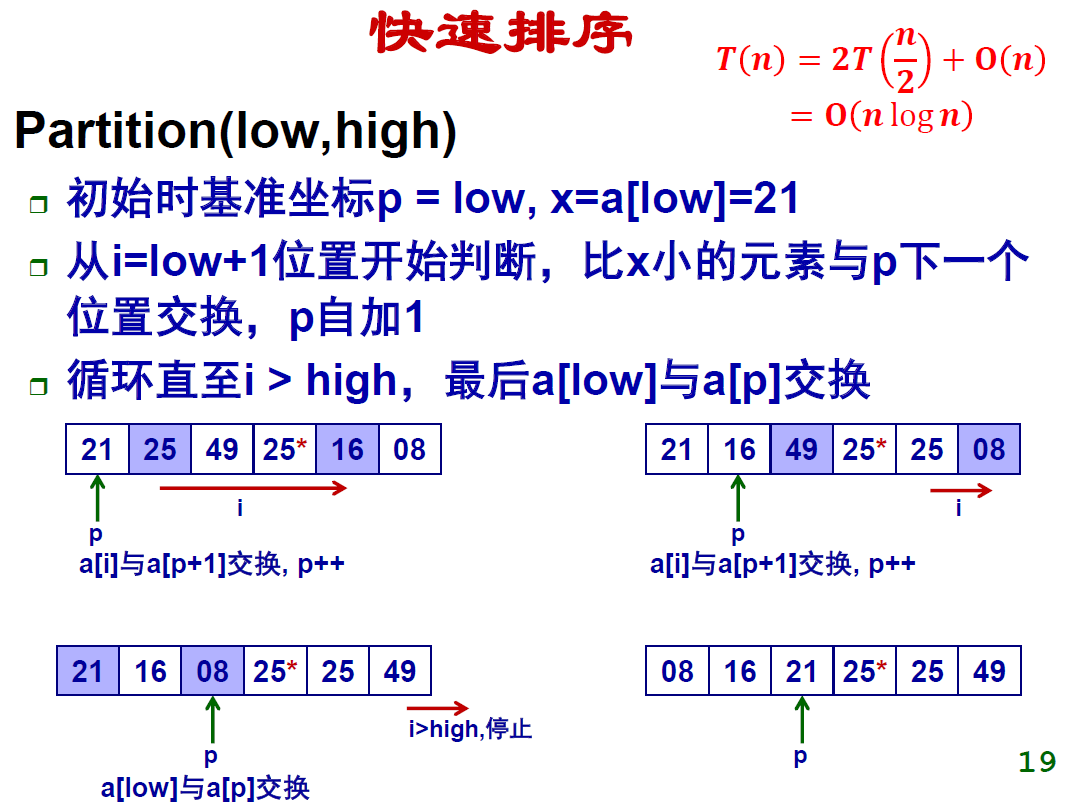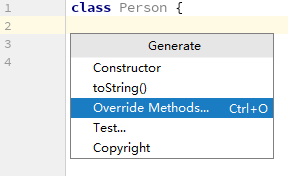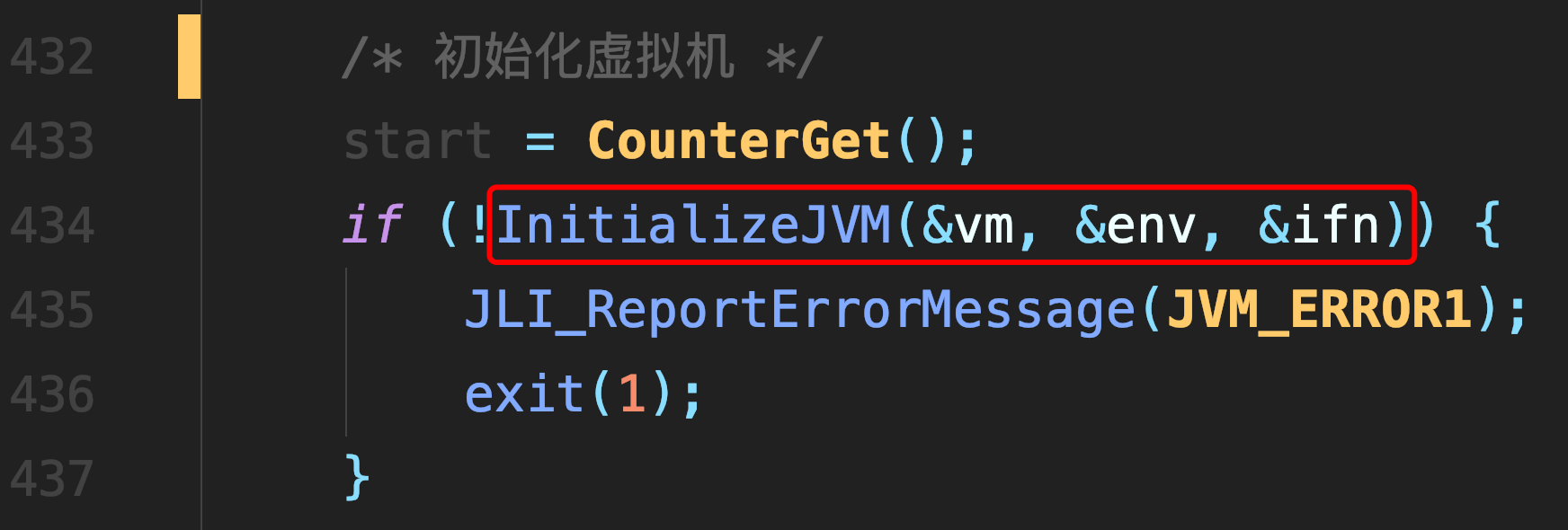# 快速排序

### 快速排序

#### 复杂度的证明

##### 最优情况##### 最坏情况#### c++代码

``````#include<iostream>
using namespace std;

int quickSort(int *a, int start, int end) {
if(start >= end) return 0;

int temp = 0;
int key = a[start];
int low = start, high = end;

while(low < high) {
while(low < high && a[high] >= key) high--;
while(low < high && a[low] <= key) low++;

if(low != high) {
temp = a[low];
a[low] = a[high];
a[high] = temp;
}
}

a[start] = a[high];
a[high] = key;
quickSort(a, start,  high - 1);
quickSort(a, high + 1, end);
return 0;
}
int main() {
int a = {1, 3, 2, 5, 8, 5, 4, 4, 0, 3};

quickSort(a, 0, 9);

for(int i = 0; i < 10; i++) printf("%d ", a[i]);
}
``````

## 另一种partition的方法

#### 这种方法更容易理解。简单的把小于基准值的元素全部交换到数组的左边python代码

``````#快速排序，另一种partition的实现

def exchange(arr, a, b):
value = arr[a]
arr[a] = arr[b]
arr[b] = value

def quickSort(arr, start, end):
if start >= end:
return

#基准坐标为start
key = arr[start]
position = start

for i in range(start+1, end+1):
#如果值比key小，则交换数组中位置i和位置position+1的两个元素的值
#并把position加1
if arr[i] < key:
exchange(arr, i, position+1)
position += 1

exchange(arr, start, position)

quickSort(arr, start, position-1)
quickSort(arr, position+1, end)

arr = [2, 3, 9, 4, 2, 4, 4, 7, 3]

quickSort(arr, 0, 8)

print (arr)
``````

##### 热门推荐
•浏览(655)
•浏览(565)
•浏览(548)
•浏览(488)
•浏览(433)
•浏览(424)
•浏览(381)
•浏览(367)
•浏览(362)
•浏览(357)